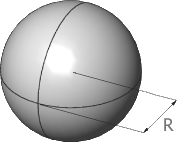# Sphere Volume Calculator

This Sphere Volume Calculator is designed to help you calculate the volume of a sphere.

To use the calculator, simply enter the radius of the sphere and click "Calculate". As you enter new dimensions into the volume calculator, a running total will also be displayed.

## Basic definition and general information about the SphereA sphere is a three-dimensional object that is perfectly round and symmetrical, with all points on its surface equidistant from its center point.

The following formula is used to calculate the volume of a sphere:

• Volume = 4/3 π * Radius3
• Where π is the constant (3.141592654)

Note: Units are provided for convenience purposes only and do not affect the calculations.

Units used for measuring the volumes of solid objects are as follows:

• cubic feet
• cubic meters
• cubic yards

Units used to measure the volumes of liquids are:

• liters
• quarts
• pints
• gallons